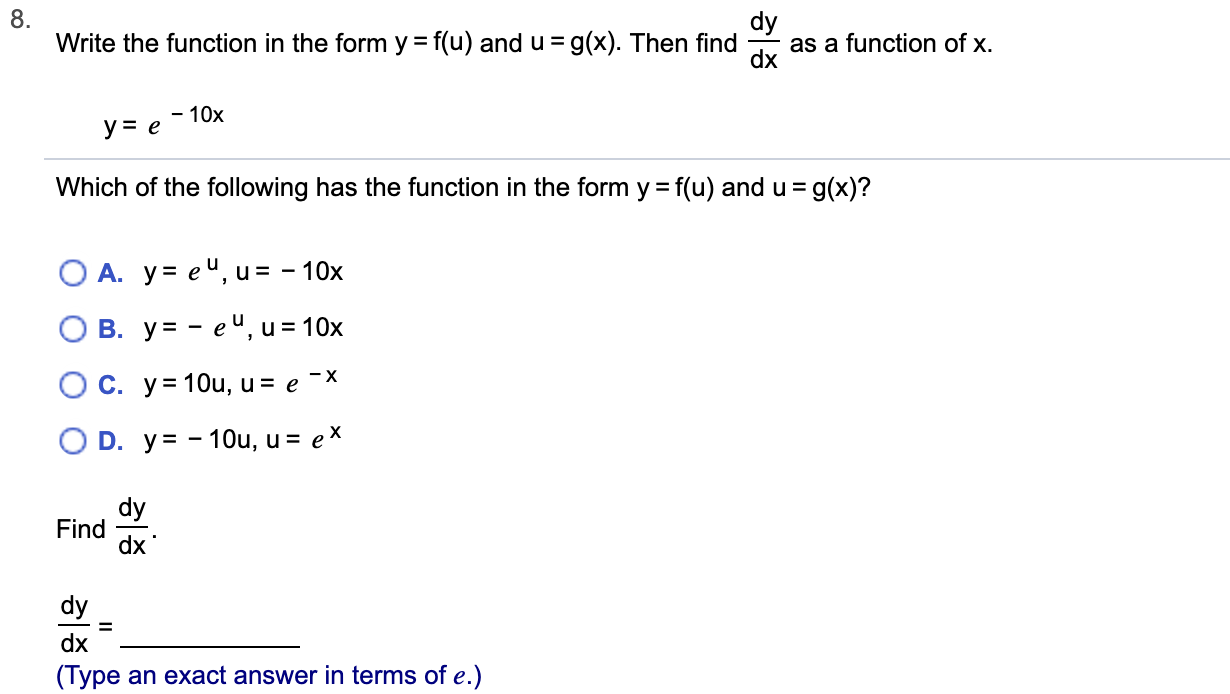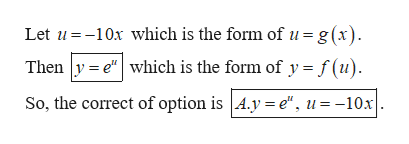# 8.Write the function in the form y = f(u) and u=g(x). Then findas a function of x.dx-10xye= g(x)?Which of the following has the function in the form y f(u) and uO A. y eu, u = -10xВ. у%3— еЧ, и%3D 10хO C. y=1Ou, u = e -XO D. y-10u, u = eXdyFinddxdydx(Type an exact answer in terms of e.)

Question
29 views

Can you help me step by step?help_outlineImage Transcriptionclose8. Write the function in the form y = f(u) and u=g(x). Then find as a function of x. dx -10x ye = g(x)? Which of the following has the function in the form y f(u) and u O A. y eu, u = -10x В. у%3— еЧ, и%3D 10х O C. y=1Ou, u = e -X O D. y-10u, u = eX dy Find dx dy dx (Type an exact answer in terms of e.) fullscreen
check_circle

Step 1

Given information:

Step 2

Write the function ...help_outlineImage TranscriptioncloseLet u10x which is the form ofu=g(x) Then y e"which is the form of y = f (u) So, the correct of option is A.y e", u=-10x fullscreen

### Want to see the full answer?

See Solution

#### Want to see this answer and more?

Solutions are written by subject experts who are available 24/7. Questions are typically answered within 1 hour.*

See Solution
*Response times may vary by subject and question.
Tagged in
MathCalculus

### Derivative